# Connector Support in ASP.NET Core Diagram

18 Jan 202324 minutes to read

Connectors are objects used to create link between two points, nodes or ports to represent the relationships between them.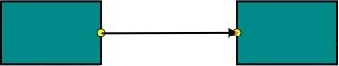## Create Connector

Connector can be created by defining the start and end points. The path to be drawn can be defined with a collection of segments.
To explore the properties of a `Connector`, refer to Connector Properties.

### Add connectors through connectors collection

The `SourcePoint` and `TargetPoint` properties of connector allow you to define the end points of a `Connector`. The following code example illustrates how to add a connector through connector collection.

• RAZOR
• ``````<ej-diagram id="diagram" width="900px" height="600px">
<e-connectors>
<e-connector name="Connector1" line-color="#606060">
<e-source-point x="100" y="100"></e-source-point>
<e-target-point x="200" y="200"></e-target-point>
</e-connector>
</e-connectors>
</ej-diagram>``````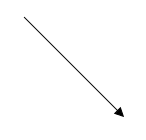### Add connector at run time

Connectors can be added at runtime with the client side method, `add`. The following code example illustrates how to add connector at runtime.

• JS
• ``````// Defines JSON
var connector = {
name: "connector",
sourcePoint: {
x: 100,
y: 100
},
targetPoint: {
x: 200,
y: 200
}
};
var diagram = \$("#DiagramContent").ejDiagram("instance");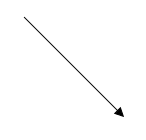### Connectors from palette

Connectors can be predefined and added to the symbol palette. You can drop those connectors into the Diagram, when required.

### Connectors through data source

Connectors are automatically generated based on the relationships defined through the data source.
The default properties for these connectors are fetched from default settings.

### Draw connectors

Connectors can be interactively drawn by clicking and dragging on the Diagram surface by using DrawingTool. For more information about drawing connectors, refer to Draw Connectors.

## Update Connector at runtime

The client side method, `updateConnector` is used to update the connectors at run time. The following code example illustrates how to update a connector at runtime.

• JS
• ``````var diagram = \$("#DiagramContent").ejDiagram("instance");
diagram.updateConnector("connectorName", {
lineColor: "#1BA0E2",
lineWidth: 5,
lineDashArray: "5,5"
});``````

## Connect nodes

The `SourceNode` and `TargetNode` properties allow to define the nodes to be connected. The following code example illustrates how to connect two nodes.

• RAZOR
• ``````<ej-diagram id="diagram" width="900px" height="600px">
<e-nodes>
<e-flow-shape name="Task 1"  offset-X="200" offset-Y="200"  shape="@FlowShapes.Process">
<e-labels>
</e-labels>
</e-flow-shape>
<e-flow-shape name="Task 2" offset-X="400" offset-Y="200"  shape="@FlowShapes.Process">
<e-labels>
</e-labels>
</e-flow-shape>
</e-nodes>
<e-connectors>
</e-connectors>
<e-default-settings>
<e-node  width="100" height="50" border-color="black" fill-color="darkCyan">
<e-labels>
<e-diagram-label font-color="white"></e-diagram-label>
</e-labels>
</e-node>
</e-default-settings>
</ej-diagram>``````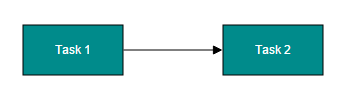NOTE

By default, connections are created at the intersecting point of segments and node bounds. The connection between any specific point of source and target nodes can be achieved with connection ports.

### Connections with ports

The `SourcePort` and `TargetPort` properties allow to create connections between some specific points of source/target nodes. The following code example illustrates how to create port to port connections.

• RAZOR
• ``````<ej-diagram id="diagram" width="900px" height="600px">
<e-nodes>
<e-flow-shape name="Task 1"  offset-X="350" offset-Y="300"  shape="@FlowShapes.Process">
<e-labels>
</e-labels>
</e-flow-shape>
<e-flow-shape name="Task 2" offset-X="200" offset-Y="250"  shape="@FlowShapes.Process">
<e-labels>
</e-labels>
<e-ports>
<e-port name="in" shape="Circle" visibility="Visible" fill-color="black">
<e-offset x="1" y="0.65f"></e-offset>
</e-port>
<e-port name="out" shape="Circle" visibility="Visible" fill-color="black">
<e-offset x="1" y="0.35f"></e-offset>
</e-port>
</e-ports>
</e-flow-shape>
<e-flow-shape name="Task 3" offset-X="350" offset-Y="200" shape="@FlowShapes.Process">
<e-labels>
</e-labels>
</e-flow-shape>
</e-nodes>
<e-connectors>
</e-connectors>
<e-default-settings>
<e-node  width="100" height="50" border-color="black" fill-color="darkCyan">
<e-labels>
<e-diagram-label font-color="white"></e-diagram-label>
</e-labels>
</e-node>
<e-connector>
<e-segments>
<e-segment type="Orthogonal"></e-segment>
</e-segments>
</e-connector>
</e-default-settings>
</ej-diagram>``````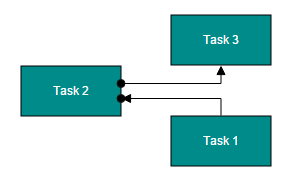## Segments

The path of the connector is defined with a collection of segments. There are three types of segments.

### Straight

Straight segment allows to create a straight line.
To create a straight line, you should specify the `Type` of the segment as “straight” and add a straight segment to `Segments` collection. The following code example illustrates how to create a default straight segment.

• RAZOR
• ``````<ej-diagram id="diagram" width="900px" height="600px">
<e-connectors>
<e-connector name="Connector1">
<e-source-point x="100" y="100"></e-source-point>
<e-target-point x="200" y="200"></e-target-point>
<e-segments>
<e-segment type="Straight"></e-segment>
</e-segments>
</e-connector>
</e-connectors>
</ej-diagram>``````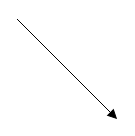The `Point` property of straight segment allows you to define the end point of it. The following code example illustrates how to define the end point of a straight segment.

• RAZOR
• ``````<ej-diagram id="diagram" width="900px" height="600px">
<e-connectors>
<e-connector name="Connector1">
<e-source-point x="100" y="100"></e-source-point>
<e-target-point x="200" y="300"></e-target-point>
<e-segments>
<e-segment type="Straight">
<e-point x="100" y="200"></e-point>
</e-segment>
</e-segments>
</e-connector>
</e-connectors>
</ej-diagram>``````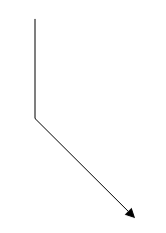### Orthogonal

Orthogonal segments are used to create segments that are perpendicular to each other.

Set the segment `Type` as “Orthogonal” to create a default orthogonal segment. The following code example illustrates how to create a default orthogonal segment.

• RAZOR
• ``````<ej-diagram id="diagram" width="900px" height="600px">
<e-connectors>
<e-connector name="Connector1">
<e-source-point x="100" y="100"></e-source-point>
<e-target-point x="200" y="200"></e-target-point>
<e-segments>
<e-segment type="Orthogonal"></e-segment>
</e-segments>
</e-connector>
</e-connectors>
</ej-diagram>``````The `Length` and `Direction` properties allow to define the flow and length of segment. The following code example illustrates how to create customized orthogonal segments.

• RAZOR
• ``````<ej-diagram id="diagram" width="900px" height="600px">
<e-connectors>
<e-connector name="Connector1">
<e-source-point x="100" y="100"></e-source-point>
<e-target-point x="200" y="200"></e-target-point>
<e-segments>
<e-segment type="Orthogonal" length="50" direction="bottom"></e-segment>
</e-segments>
</e-connector>
</e-connectors>
</ej-diagram>``````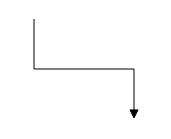#### Avoid overlapping

Orthogonal segments are automatically re-routed, in order to avoid overlapping with the source and target nodes. The following images illustrate how orthogonal segments are re-routed.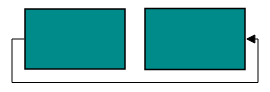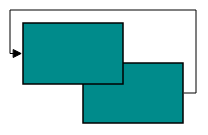NOTE

Overlapping with source and target nodes are only avoided. Other nodes are not considered as obstacles.

### Bezier

Bezier segments are used to create curve segments and the curves are configurable either with the control points or with vectors.

To create a bezier segment, the `Segment.Type` is set as `Bezier`. The following code example illustrates how to create a default Bezier segment.

• RAZOR
• ``````<ej-diagram id="diagram" width="900px" height="600px">
<e-connectors>
<e-connector name="Connector1">
<e-source-point x="100" y="100"></e-source-point>
<e-target-point x="200" y="200"></e-target-point>
<e-segments>
<e-segment type="Bezier"></e-segment>
</e-segments>
</e-connector>
</e-connectors>
</ej-diagram>``````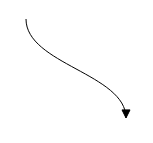The `Point1` and `Point2` properties of bezier segment enable you to set the control points. The following code example illustrates how to configure the Bezier segments with control points.

• RAZOR
• ``````<ej-diagram id="diagram" width="900px" height="600px">
<e-connectors>
<e-connector name="Connector1">
<e-source-point x="100" y="200"></e-source-point>
<e-target-point x="250" y="200"></e-target-point>
<e-segments>
<e-segment type="Bezier">
<e-point1 x="125" y="75"></e-point1>
<e-point2 x="225" y="75"></e-point2>
</e-segment>
</e-segments>
</e-connector>
</e-connectors>
</ej-diagram>``````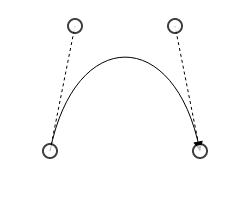The `Vector1` and `Vector2` properties of bezier segment enable you to define the vectors. The following code illustrates how to configure a bezier curve with vectors.

• RAZOR
• ``````<ej-diagram id="diagram" width="900px" height="600px">
<e-connectors>
<e-connector name="Connector1">
<e-source-point x="100" y="200"></e-source-point>
<e-target-point x="250" y="200"></e-target-point>
<e-segments>
<e-segment type="Bezier">
<e-vector1 angle="270" distance="75"></e-vector1>
<e-vector2 angle="270" distance="75"></e-vector2>
</e-segment>
</e-segments>
</e-connector>
</e-connectors>
</ej-diagram>``````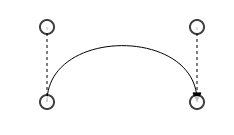### Complex segments

Multiple segments can be defined one after another. To create a connector with multiple segments, define and add the segments to `Connector.Segments` collection. The Following code example illustrates how to create a connector with multiple segments.

• RAZOR
• ``````<ej-diagram id="diagram" width="900px" height="600px">
<e-connectors>
<e-connector name="Connector1">
<e-source-point x="100" y="200"></e-source-point>
<e-target-point x="250" y="300"></e-target-point>
<e-segments>
<e-segment type="Orthogonal" length="150" direction="bottom"></e-segment>
<e-segment type="Orthogonal" length="150" direction="right"></e-segment>
</e-segments>
</e-connector>
</e-connectors>
</ej-diagram>``````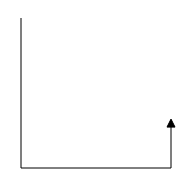## Decorator

Start and end points of a connector can be decorated with some customizable shapes like arrows, circles, diamond or path. You can decorate the connection end points with the `SourceDecorator` and `TargetDecorator` properties of connector.
To explore the properties of decorators, refer to Decorator Properties.

The `Shape` property of decorator allows to define the shape of the decorators. The following code example illustrates how to create decorators of various shapes.

• RAZOR
• ``````<ej-diagram id="diagram" width="900px" height="600px">
<e-connectors>
<e-connector name="Connector1">
<e-source-point x="100" y="100"></e-source-point>
<e-target-point x="200" y="200"></e-target-point>
<e-source-decorator shape="Circle" width="10" height="10"></e-source-decorator>
<e-target-decorator shape="Arrow"  width="10" height="10"></e-target-decorator>
</e-connector>
<e-connector name="Connector2">
<e-source-point x="300" y="100"></e-source-point>
<e-target-point x="400" y="200"></e-target-point>
<e-source-decorator shape="Diamond" width="10" height="10"></e-source-decorator>
<e-target-decorator shape="OpenArrow" width="10" height="10"></e-target-decorator>
</e-connector>
<e-connector name="Connector3">
<e-source-point x="500" y="100"></e-source-point>
<e-target-point x="600" y="200"></e-target-point>
<e-target-decorator shape="Path" path-data="M 376.892,225.284L 371.279,211.95L 376.892,198.617L 350.225,211.95L 376.892,225.284 Z"></e-target-decorator>
</e-connector>
</e-connectors>
</ej-diagram>``````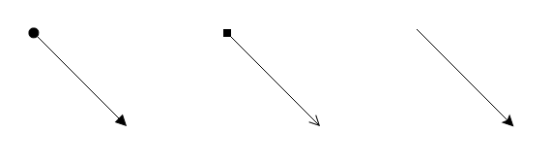Padding is used to leave space between the Connector’s end point and the object to where it is connected.

The `SourcePadding` and `TargetPadding` properties of connector define the space to be left between the connection end points and the source and target nodes of connector. The following code example illustrates how to leave space between the connection end points and source, target nodes.

• RAZOR
• ``````<ej-diagram id="diagram" width="900px" height="600px">
<e-nodes>
<e-flow-shape name="Task 1" offset-x="200" offset-y="200" shape="@FlowShapes.Process">
<e-labels>
</e-labels>
</e-flow-shape>
<e-flow-shape name="Task 2" offset-x="400" offset-y="200" shape="@FlowShapes.Process">
<e-labels>
</e-labels>
</e-flow-shape>
</e-nodes>
<e-connectors>
</e-connectors>
<e-default-settings>
<e-node width="100" height="50" border-color="black" fill-color="darkCyan">
<e-labels>
<e-diagram-label font-color="white"></e-diagram-label>
</e-labels>
</e-node>
</e-default-settings>
</ej-diagram>``````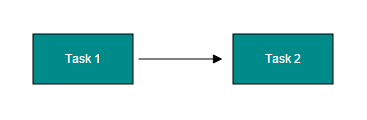The `ConnectorPadding` property of node defines the space to be left between the node bounds and its edges. The following code example illustrates how to leave the space between a node and its connections.

• RAZOR
• ``````<ej-diagram id="diagram" width="900px" height="600px">
<e-nodes>
<e-labels>
</e-labels>
</e-flow-shape>
<e-flow-shape name="Task 2" offset-x="400" offset-y="200" shape="@FlowShapes.Process">
<e-labels>
</e-labels>
</e-flow-shape>
</e-nodes>
<e-connectors>
</e-connectors>
<e-default-settings>
<e-node width="100" height="50" border-color="black" fill-color="darkCyan">
<e-labels>
<e-diagram-label font-color="white"></e-diagram-label>
</e-labels>
</e-node>
</e-default-settings>
</ej-diagram>``````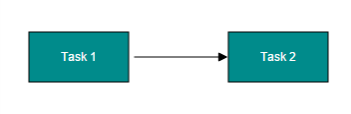The `ConnectorPadding` property of port defines the space between the ports and its in/out edges. The following code example illustrates how to leave the space between ports and its connections.

• RAZOR
• ``````<ej-diagram id="diagram" width="900px" height="600px">
<e-nodes>
<e-flow-shape name="Task 1" offset-x="200" offset-y="200" shape="@FlowShapes.Process">
<e-labels>
</e-labels>
</e-flow-shape>
<e-flow-shape name="Task 2" offset-x="400" offset-y="200" shape="@FlowShapes.Process">
<e-labels>
</e-labels>
<e-ports>
<e-port name="port" shape="Circle" visibility="Visible" fill-color="black" connector-padding="5">
<e-offset x="0" y="0.5f"></e-offset>
</e-port>
</e-ports>
</e-flow-shape>
</e-nodes>
<e-connectors>
</e-connectors>
<e-default-settings>
<e-node width="100" height="50" border-color="black" fill-color="darkCyan">
<e-labels>
<e-diagram-label font-color="white"></e-diagram-label>
</e-labels>
</e-node>
</e-default-settings>
</ej-diagram>``````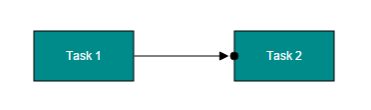## Bridging

Line Bridging creates a bridge for lines to smartly cross over other lines, at points of intersection. When two line connectors meet each other, the line with the higher z-order (upper one) draws an arc over the underlying connector.
Bridging can be enabled/disabled either with the `Connector.Constraints` or `Diagram.Constraints`. The following code example illustrates how to enable line bridging.

• RAZOR
• ``````<ej-diagram id="diagram" width="900px" height="600px">
<e-connectors>
<e-connector name="Connector1" constraints="Default & ~ConnectorConstraints.InheritBridging | ConnectorConstraints.Bridging">
<e-source-point x="100" y="100"></e-source-point>
<e-target-point x="200" y="200"></e-target-point>
</e-connector>
</e-connectors>
</ej-diagram>``````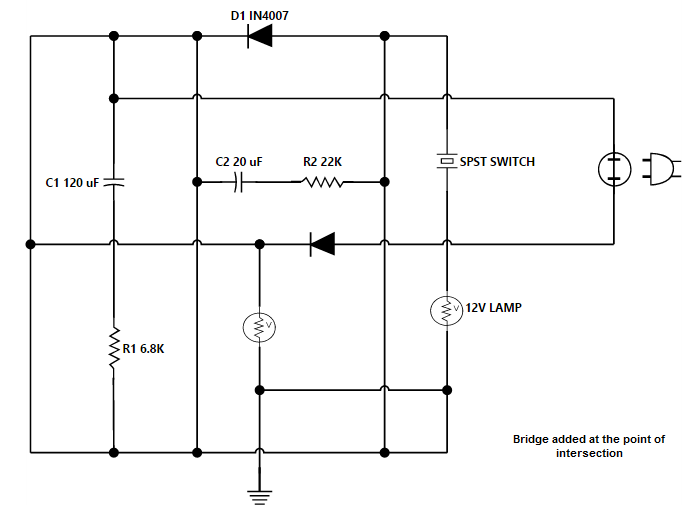The direction of the bridge can be customized with the property `BridgeDirection`. BridgeDirection defines the intersecting segment where the bridge has to be inserted. By default, the bridge direction points to the top.

To explore the bridge directions, refer to Bridge Directions.

The following code example illustrates how to draw the bridge at the bottom direction.

• RAZOR
• ``<ej-diagram id="diagram" width="900px" height="600px" constraints="Default | DiagramConstraints.Bridging" bridge-direction="Bottom"></ej-diagram>``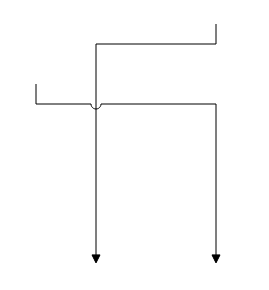Limitation: Bezier segments do not support bridging.

Corner radius allows to create connectors with rounded corners. The radius of the rounded corner is set with `CornerRadius` property.

• RAZOR
• ``````<ej-diagram id="diagram" width="900px" height="600px">
<e-nodes>
<e-flow-shape name="Task 1" offset-x="200" offset-y="200" shape="@FlowShapes.Process">
<e-labels>
</e-labels>
</e-flow-shape>
<e-flow-shape name="Task 2" offset-x="350" offset-y="300" shape="@FlowShapes.Process">
<e-labels>
</e-labels>
</e-flow-shape>
</e-nodes>
<e-connectors>
</e-connectors>
<e-default-settings>
<e-node width="100" height="50" border-color="black" fill-color="darkCyan">
<e-labels>
<e-diagram-label font-color="white"></e-diagram-label>
</e-labels>
</e-node>
<e-connector>
<e-segments>
<e-segment type="Orthogonal"></e-segment>
</e-segments>
</e-connector>
</e-default-settings>
</ej-diagram>``````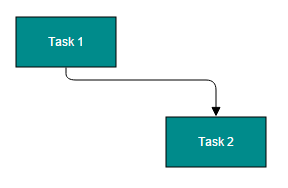## Appearance

Stroke width, stroke color, and style of the lines and decorators can be customized with a set of defined properties.

### Segment Appearance

The following code example illustrates how to customize the segment appearance.

• RAZOR
• ``````<ej-diagram id="diagram" width="900px" height="600px">
<e-connectors>
<e-connector name="Connector1" line-width="2" line-color="green" line-dash-array="2,2" opacity="0.8f">
<e-source-point x="100" y="100"></e-source-point>
<e-target-point x="200" y="200"></e-target-point>
<e-segments>
<e-segment type="Orthogonal"></e-segment>
</e-segments>
</e-connector>
</e-connectors>
</ej-diagram>``````

### Decorator Appearance

The following code example illustrates how to customize the appearance of the decorator.

• RAZOR
• ``````<ej-diagram id="diagram" width="900px" height="600px">
<e-connectors>
<e-connector name="Connector1" line-width="2" line-color="green" line-dash-array="2,2" opacity="0.8f">
<e-source-point x="100" y="100"></e-source-point>
<e-target-point x="200" y="200"></e-target-point>
<e-segments>
<e-segment type="Orthogonal"></e-segment>
</e-segments>
<e-target-decorator shape="Arrow" fill-color="red" border-color="green" border-width="2" width="10" height="10"></e-target-decorator>
</e-connector>
</e-connectors>
</ej-diagram>``````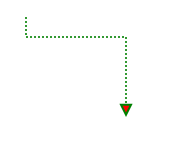## Interaction

Diagram allows to edit the connectors at runtime. To edit the connector segments at runtime, refer to Connection Editing.

## Constraints

The `Constraints` property of connector allows to enable/disable certain features of connectors. For more information about constraints, refer to Connector Constraints.